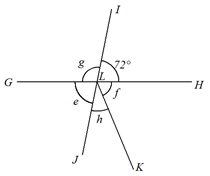### Sample Problem

The figure is not drawn to scale. IJ and GH are straight lines.

Given that∠e + ∠f = 150°. Find∠e, ∠f, ∠g, ∠h.∠e = °, ∠f = °, ∠g = °, ∠h = °.

#### Solution

∠e and ∠ILH are vertically opposite angles.

∠e = ∠ILH = 72°.

∠f + ∠e = 150°.

∠f = 150° − ∠e = 150° − 72° = 78°.

∠e, ∠f and ∠h are angles on a straight line. Their sum is 180°.

∠e + ∠f + ∠h = 180°. ∠e + ∠f = 150°.

∠h = 180° − (∠e + ∠f) = 180° − 150° = 30°.

∠e and ∠g are angles on a straight line. Their sum is 180°.

∠e + ∠g = 180°

∠g = 180° − ∠e = 180° − 72° = 108°.